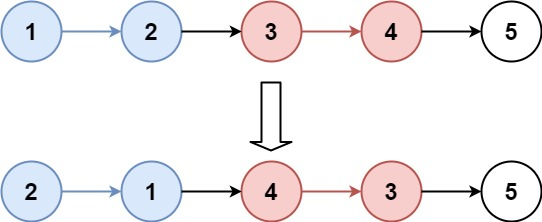## 25. Reverse Nodes in k-Group

Given a linked list, reverse the nodes of a linked list k at a time and return its modified list.

k is a positive integer and is less than or equal to the length of the linked list. If the number of nodes is not a multiple of k then left-out nodes, in the end, should remain as it is.

• Could you solve the problem in `O(1)` extra memory space?
• You may not alter the values in the list's nodes, only nodes itself may be changed.

Example 1:```Input: head = [1,2,3,4,5], k = 2
Output: [2,1,4,3,5]
```

Example 2:```Input: head = [1,2,3,4,5], k = 3
Output: [3,2,1,4,5]
```

Example 3:

```Input: head = [1,2,3,4,5], k = 1
Output: [1,2,3,4,5]
```

Example 4:

```Input: head = , k = 1
Output: 
```

Constraints:

• The number of nodes in the list is in the range `sz`.
• `1 <= sz <= 5000`
• `0 <= Node.val <= 1000`
• `1 <= k <= sz`

## Rust Solution

``````struct Solution;
use rustgym_util::*;
use std::collections::VecDeque;

impl Solution {
let mut count = 0;
let mut queue: VecDeque<ListLink> = VecDeque::new();
let k = k as usize;
while let Some(mut node) = p {
p = node.next.take();
queue.push_back(Some(node));
count += 1;
if count == k {
break;
}
}
if queue.len() == k {
let mut prev: ListLink = Self::reverse_k_group(p, k as i32);
while let Some(link) = queue.pop_front() {
if let Some(mut node) = link {
node.next = prev;
prev = Some(node);
}
}
prev
} else {
let mut prev: ListLink = None;
while let Some(link) = queue.pop_back() {
if let Some(mut node) = link {
node.next = prev;
prev = Some(node);
}
}
prev
}
}
}

#[test]
fn test() {
let head = list!(1, 2, 3, 4, 5);
let k = 2;
let res = list!(2, 1, 4, 3, 5);